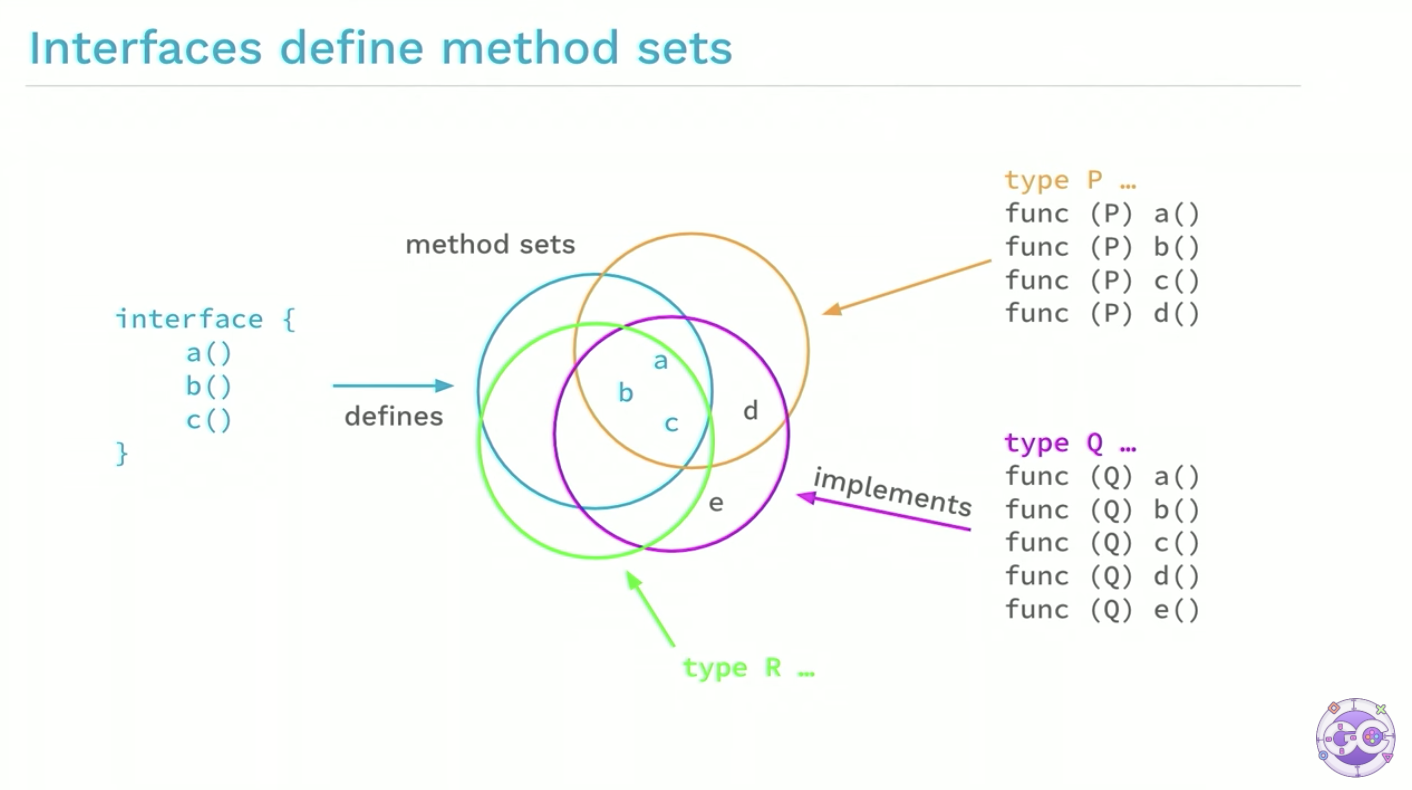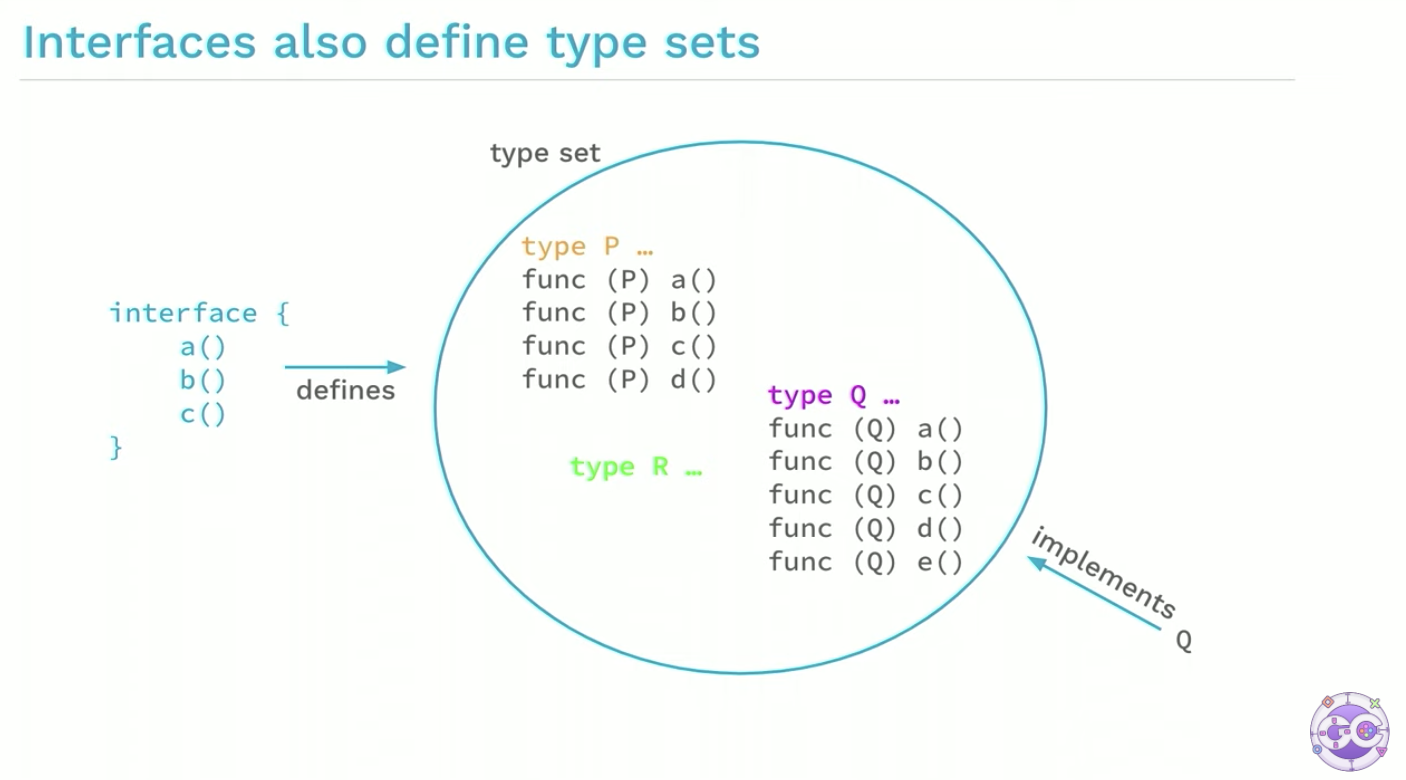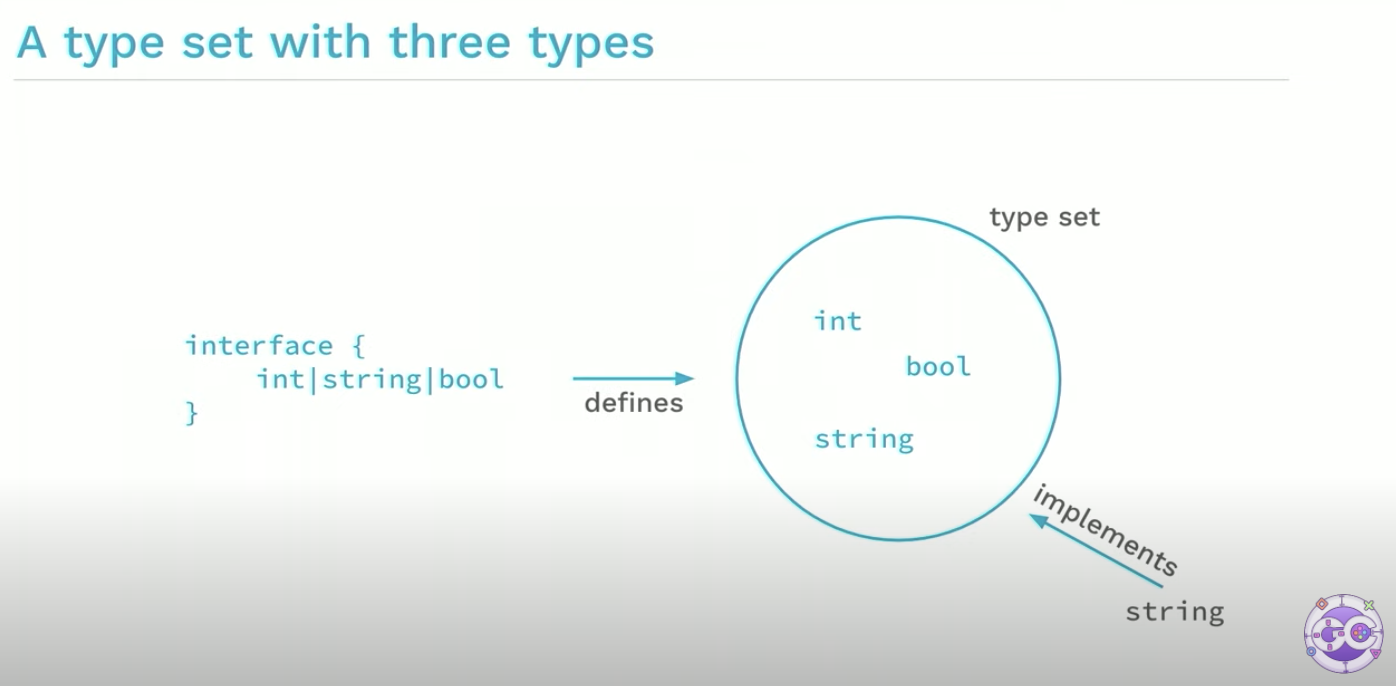Go1.18 关于泛型部分，主要有三个特性：

• Type parameters for functions and types，即函数和类型的类型参数
• Type sets defined by interfaces，即由接口定义的类型集合
• Type inference，即类型推断

## 1、类型参数

### 类型参数列表（Type parameter lists）

``````[P, Q constraint1, R constraint2]
``````

``````func min(x, y float64) float64 {
if x < y {
return x
}
return y
}
``````

``````func min[T constraints.Ordered](x, y T) T {
if x < y {
return x
}
return y
}
``````

• 多了一个 `[T constraints.Ordered]`，这就是类型参数列表，声明了一个类型 T，它的约束是 constraints.Ordered，即类型 T 满足它规定的条件
• 参数类型 float64 变成了 T，而不是具体的某个类型

``````m := min[int](2, 3)
``````

### 实例化

1）用类型实参（type arguments）替换类型形参（type parameters）

2）检查类型实参（type arguments）是否实现了类型约束

``````fmin := min[float64]
m := fmin(2.3, 3.4)

// 和下面等价
m := min[float64](2.3, 3.4)
// 相当于 m := (min[float64])(2.3, 3.4)
``````

### 类型的类型参数

``````type Tree[T interface{}] struct {
left, right *Tree[T]
data        T
}

func (t *Tree[T]) Lookup(x T) *Tree[T]

var stringTree Tree[string]
``````

## 2、类型集合（Type sets）

``````func min[T constraints.Ordered](x, y T) T
``````

constraints.Ordered 是 Go1.18 内置的一个类型约束，它有两个功能：

• 只有值支持排序的类型才能传递给类型参数 T；
• T 类型的值必须支持 `<` 操作符，因为函数体中使用了该操作符。

### 类型约束是接口``````type Xxx interface {
int | string | bool
}
``````

``````// Ordered is a constraint that permits any ordered type: any type
// that supports the operators < <= >= >.
// If future releases of Go add new ordered types,
// this constraint will be modified to include them.
type Ordered interface {
Integer | Float | ~string
}
``````

Ordered 定义了所有 interger、浮点数和字符串类型的集合。所以，< 操作符也是支持的。这其中的 Integer、Float 也在 constraints 包有定义。

``````[S interface{~[]E}, E interface{}]
``````

``````[S ~[]E, E interface{}]
``````

Go1.18 中，any 是 interface{} 的别名，因此可以进一步写为：

``````[S ~[]E, E any]
``````

E 是切片的元素类型，`~[]E` 表示底层是 `[]E` 切片类型的都符合该约束。

## 3、类型推断

``````var a, b, m float64
m := min[float64](a, b)
``````

``````func Scale[E constraints.Integer](s []E, c E) []E {
r := make([]E, len(s))
for i, v := range s {
r[i] = v * c
}
return r
}
``````

``````type Point []int32

func (p Point) String() string {
// 实现细节不重要，忽略
return "point"
}
``````

``````func ScaleAndPrint(p Point) {
r := Scale(p, 2)
fmt.Println(r.String())
}
``````

``````func main() {
p := Point{3, 2, 4}
ScaleAndPrint(p)
}
``````

``````func Scale[S ~[]E, E constraints.Integer](s S, c E) S {
r := make(S, len(s))
for i, v := range s {
r[i] = v * c
}
return r
}
``````

``````package main

import (
"constraints"
"fmt"
)

func Scale[S ~[]E, E constraints.Integer](s S, c E) S {
r := make(S, len(s))
for i, v := range s {
r[i] = v * c
}
return r
}

type Point []int32

func (p Point) String() string {
// 实现细节不重要，忽略
return "point"
}

func ScaleAndPrint(p Point) {
r := Scale(p, 2)
fmt.Println(r.String())
}

func main() {
p := Point{3, 2, 4}
ScaleAndPrint(p)
}
``````

## 4、什么时候用泛型

• 对于 slice、map、channel 等类型，如果它们的元素类型是不确定的，操作这类类型的函数可以考虑用泛型
• 一些通用目的的数据结构，比如前面提到的二叉树等
• 如果一些函数行为相同，只是类型不同，可以考虑用泛型重构

``````func ReadFour[T io.Reader](r T) ([]byte, error)
``````

``````func ReadFour(r io.Reader) ([]byte, error)
``````

## 5、总结2837 字

2022-02-03 22:30 +0800